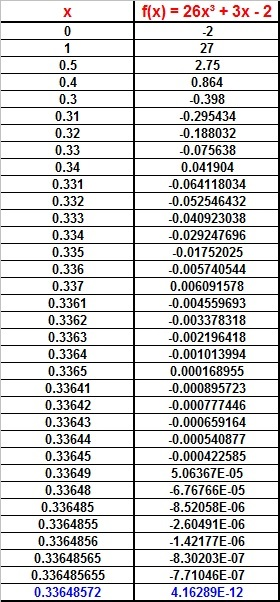Showing posts with label Chemical Engineering Math. Show all posts
Showing posts with label Chemical Engineering Math. Show all posts

## Monday, June 22, 2015

### Chemical Equilibrium of Gases, 2

Category: Chemical Engineering Math

"Published in Newark, California, USA"

At 3000K and 1 atm, CO2 is 40% dissociated to CO and O2. Calculate its percentage dissociation when the pressure is increased to 2 atm.

Solution:

Consider the chemical reaction above as follows

For gases, the equilibrium constant of the above reaction is

At the start of the reaction, the total pressure of CO2 which is the total pressure of gas mixture is equal to1 atm. At equilibrium, the partial pressure of gases are as follows:

Hence, the equilibrium constant for the reaction above is

For 1 atm, if x is the partial pressure of CO2 dissociated, then the partial pressure of gases are as follows:

If the pressure is increased to 2 atm, then the partial pressure of gases will be multiplied by 2. Substitute the value of partial pressure of gases at the chemical equilibrium equation above, we have

Since the above equation cannot be factored by factoring and even synthetic division, then we have to do the trial and error method until we get zero at the right side of the equation. The best way is to use Excel program in solving for the value of x because we can input the formula and we can assign any values of x in the spreadsheet as followsPhoto by Math Principles in Everyday Life

From the Excel spreadsheet, the value of x which is the partial pressure of CO2 dissociated is 0.33648572 atm since the right side of the equation is very close to zero.

Therefore, the percent of dissociation of CO2 is

## Sunday, June 21, 2015

### Ideal Gas Law Problems, 6

Category: Chemical Engineering Math

"Published in Vacaville, California, USA"

PCl5(g) dissociates into PCl3(g) and Cl2(g) when heated at 250°C and 1 atm. If the density of the gas mixture at equilibrium is 4.4 g/L, what is the fraction of PCl5(g) dissociated?

Solution:

Consider the chemical reactions above as follows

At equilibrium, there are PCl5(g), PCl3(g) and Cl2(g) gas mixture in 1 liter. If the volume of the reaction at equilibrium is 1 liter, then the number of moles of gas mixture at equilibrium is

At the start of the reaction, the volume is also 1 liter. The number of moles of PCl5(g) which is also the number of moles of gas mixture at the start of the reaction is

Hence, the amount of PCl5(g) dissociated or converted into PCl3(g) and Cl2(g) is

Therefore, the fraction of PCl5(g) dissociated is

## Saturday, June 20, 2015

### Dalton's Law of Partial Pressure, 4

Category: Chemical Engineering Math

"Published in Newark, California, USA"

A solvent-water mixture is to be distilled at 95°C. The vapor pressure of the solvent at this temperature is 130 mm Hg and that of water is 640 mm Hg. The solvent is immiscible in water and has a molecular weight of 150. What is the weight of the solvent in kilograms that will be carried over in the distillate with 200 kg of water?

Solution:

From the given word problem, it is about Dalton's Law of Partial Pressure because it involves the mixture of gas vapors which are solvent and water. In this problem, the molecular weight of a solvent is unknown but the partial pressure of solvent and water vapor are given. From the given partial pressures, we can solve for the mole fraction of a solvent vapor as follows

If W is the weight of a solvent and its molecular weight is 150 kg/kgmole, therefore the weight of a solvent that will be carried over in the distillate with 200 kg water is

## Friday, June 19, 2015

### Dalton's Law of Partial Pressure, 3

Category: Chemical Engineering Math

"Published in Newark, California, USA"

If ethanol and methanol are mixed 50/50 by weight at 60°C, and the solution assumed ideal, what is the composition of the vapor above the solution?

Solution:

From the given word problem, it is about Dalton's Law of Partial Pressure because it involves the mixture of gas vapors. Ethanol and methanol are mixed in equal proportion or amount.

Basis: 100 grams of the mixture

Let's consider first the liquid phase. The number of moles of ethanol and methanol in the solution are

Next, consider the gas or vapor phase. From Saturated Vapor Pressure Data, the vapor pressure of pure ethanol at 60°C is 0.461395 atm and for the pure methanol is 0.832997 atm. Hence, the partial pressure of ethanol is

and the partial pressure of methanol is

Therefore, the % ethanol in the vapor is

and the % methanol in the vapor is